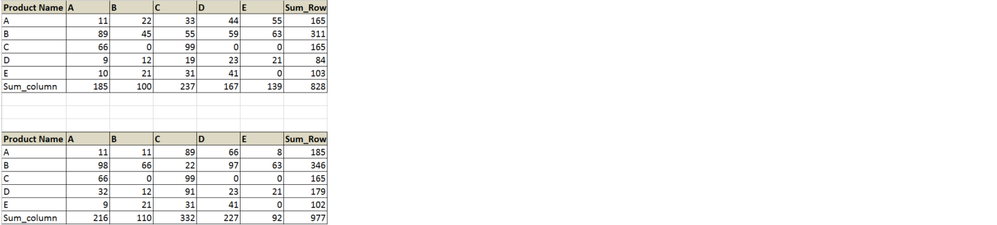## Proc IML- Matrix element operation

Hi Guys,

I'm new to IML and need some help to start with. hope someone will help.

Below ,are the two matrix which i have. I want a new matrix, by dividing the Sum_row values of second matrix with each element of the first. So, the output matrix first row should be:

A 11/185   22/185 33/185   44/185  55/185

B 89/346   45/346   55/346  59/346  63/346

... so on and so forth.  Any help, will really be appreciated.1 ACCEPTED SOLUTION

Accepted SolutionsKsharp
Super User

## Re: Proc IML- Matrix element operation

``````data a;
input a b c d e;
cards;
11 22 33 44 55
89 45 55 59 63
66  0 99  0  0
66  0 99  0  0
66  0 99  0  0
;
run;

data b;
input a b c d e;
cards;
11 22 33 44 55
89 45 55 59 63
66  0 99  0  0
66  0 99  0  0
66  0 99  0  0
;
run;

proc iml;
use a;
read all var _num_ into x[c=vname];
close;
use b;
read all var _num_ into y;
close;

colSums = y[+, ];
want=x/colSums ;

create want from want[c=vname r=vname];
append from want[r=vname];
close;
quit;

proc print noobs;run;``````
4 REPLIES 4

## Re: Proc IML- Matrix element operation

SAS/IML supports a shorthand notation for common operations on rows and columns, including sums, products, sums of squares, min, max, and means. Collectively, these are known as "subscript reduction operators." You can read about them at

"Use subscript reduction operations."

``````
proc iml;
M = {11 22 33 44 55,
89 45 55 59 63,
66  0 99  0  0
/* etc */   };
colSums = M[+, ];
print colSums;P = M / colSums;``````Ksharp
Super User

## Re: Proc IML- Matrix element operation

``````proc iml;
first={11 22 33 44 55,
89 45 55 59 63,
66  0 99  0  0
/* etc */   };

second = {11 22 33 44 55,
89 45 55 59 63,
66  0 99  0  0
/* etc */   };
colSums = M[+, ];
print colSums;

want=first/colSums ;
print want;
quit;``````

## Re: Proc IML- Matrix element operation

Hi Ksharp,

Thanks for your reply. Its all good and its all working fine. However, it only gives me the numeric values. I want all the variables together.

Output i receive is as follows:

 A B C D E ROW1 Value Value Value Value Value ROW2 Value Value Value Value Value ROW3 Value Value Value Value Value ROW4 Value Value Value Value Value ROW5 Value Value Value Value Value

P.S. the value represents the resultant value post division.( as per my expectation)

But, i need  below as output, i.e. the character value(Product name) columwise and calculated values in the rows.

 Product Name A B C D E A Value Value Value Value Value B Value Value Value Value Value C Value Value Value Value Value D Value Value Value Value Value E Value Value Value Value Value

Massive thanks for your support Sir.Ksharp
Super User

## Re: Proc IML- Matrix element operation

``````data a;
input a b c d e;
cards;
11 22 33 44 55
89 45 55 59 63
66  0 99  0  0
66  0 99  0  0
66  0 99  0  0
;
run;

data b;
input a b c d e;
cards;
11 22 33 44 55
89 45 55 59 63
66  0 99  0  0
66  0 99  0  0
66  0 99  0  0
;
run;

proc iml;
use a;
read all var _num_ into x[c=vname];
close;
use b;
read all var _num_ into y;
close;

colSums = y[+, ];
want=x/colSums ;

create want from want[c=vname r=vname];
append from want[r=vname];
close;
quit;

proc print noobs;run;``````
From The DO Loop